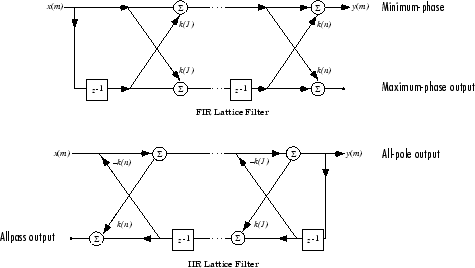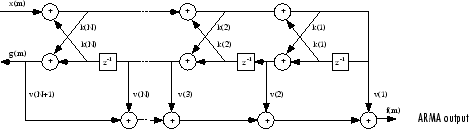Documentation

## Discrete-Time System Models

The discrete-time system models are representational schemes for digital filters. The MATLAB® technical computing environment supports several discrete-time system models, which are described in the following sections:

### Transfer Function

The transfer function is a basic Z-domain representation of a digital filter, expressing the filter as a ratio of two polynomials. It is the principal discrete-time model for this toolbox. The transfer function model description for the Z-transform of a digital filter's difference equation is

`$Y\left(z\right)=\frac{b\left(1\right)+b\left(2\right){z}^{-1}+\dots +b\left(n+1\right){z}^{-n}}{a\left(1\right)+a\left(2\right){z}^{-1}+\dots +a\left(m+1\right){z}^{-m}}X\left(z\right).$`

Here, the constants b(i) and a(i) are the filter coefficients, and the order of the filter is the maximum of n and m. In the MATLAB environment, you store these coefficients in two vectors (row vectors by convention), one row vector for the numerator and one for the denominator. See Filters and Transfer Functions for more details on the transfer function form.

### Zero-Pole-Gain

The factored or zero-pole-gain form of a transfer function is

`$H\left(z\right)=\frac{q\left(z\right)}{p\left(z\right)}=k\frac{\left(z-q\left(1\right)\right)\left(z-q\left(2\right)\right)...\left(z-q\left(n\right)\right)}{\left(z-p\left(1\right)\right)\left(z-p\left(2\right)\right)...\left(z-p\left(n\right)\right)}.$`

By convention, polynomial coefficients are stored in row vectors and polynomial roots in column vectors. In zero-pole-gain form, therefore, the zero and pole locations for the numerator and denominator of a transfer function reside in column vectors. The factored transfer function gain k is a MATLAB scalar.

The `poly` and `roots` functions convert between polynomial and zero-pole-gain representations. For example, a simple IIR filter is

```b = [2 3 4]; a = [1 3 3 1]; ```

The zeros and poles of this filter are

```q = roots(b) p = roots(a) % Gain factor k = b(1)/a(1) ```

Returning to the original polynomials,

```bb = k*poly(q) aa = poly(p) ```

Note that `b` and `a` in this case represent the transfer function:

`$H\left(z\right)=\frac{2+3{z}^{-1}+4{z}^{-2}}{1+3{z}^{-1}+3{z}^{-2}+{z}^{-3}}=\frac{z\text{\hspace{0.17em}}\left(2{z}^{2}+3z+4\right)}{{z}^{3}+3{z}^{2}+3z+1}.$`

For `b = [2 3 4]`, the `roots` function misses the zero for z equal to 0. In fact, the function misses poles and zeros for z equal to 0 whenever the input transfer function has more poles than zeros, or vice versa. This is acceptable in most cases. To circumvent the problem, however, simply append zeros to make the vectors the same length before using the `roots` function; for example, `b = [b 0]`.

### State Space

It is always possible to represent a digital filter, or a system of difference equations, as a set of first-order difference equations. In matrix or state-space form, you can write the equations as

`$\begin{array}{c}x\left(n+1\right)=Ax\left(n\right)+Bu\left(n\right)\\ y\left(n\right)\text{ }\text{ }\text{ }\text{ }\text{ }\text{ }\text{ }\text{ }\text{ }\text{ }\text{ }\text{ }\text{ }\text{ }\text{ }=Cx\left(n\right)+Du\left(n\right),\end{array}$`

where `u` is the input, `x` is the state vector, and `y` is the output. For single-channel systems, `A` is an `m`-by-`m` matrix where `m` is the order of the filter, `B` is a column vector, `C` is a row vector, and `D` is a scalar. State-space notation is especially convenient for multichannel systems where input `u` and output `y` become vectors, and `B`, `C`, and `D` become matrices.

State-space representation extends easily to the MATLAB environment. `A`, `B`, `C`, and `D` are rectangular arrays; MATLAB functions treat them as individual variables.

Taking the Z-transform of the state-space equations and combining them shows the equivalence of state-space and transfer function forms:

Don't be concerned if you are not familiar with the state-space representation of linear systems. Some of the filter design algorithms use state-space form internally but do not require any knowledge of state-space concepts to use them successfully. If your applications use state-space based signal processing extensively, however, see the Control System Toolbox™ product for a comprehensive library of state-space tools.

### Partial Fraction Expansion (Residue Form)

Each transfer function also has a corresponding partial fraction expansion or residue form representation, given by

`$\frac{b\left(z\right)}{a\left(z\right)}=\frac{r\left(1\right)}{1-p\left(1\right){z}^{-1}}+...+\frac{r\left(n\right)}{1-p\left(n\right){z}^{-1}}+k\left(1\right)+k\left(2\right){z}^{-1}+...+k\left(m-n+1\right){z}^{-\left(m-n\right)}$`

provided H(z) has no repeated poles. Here, n is the degree of the denominator polynomial of the rational transfer function b(z)/a(z). If r is a pole of multiplicity sr, then H(z) has terms of the form:

`$\frac{r\left(j\right)}{1-p\left(j\right){z}^{-1}}+\frac{r\left(j+1\right)}{{\left(1-p\left(j\right){z}^{-1}\right)}^{2}}...+\frac{r\left(j+{s}_{r}-1\right)}{{\left(1-p\left(j\right){z}^{-1}\right)}^{{s}_{r}}}$`

The Signal Processing Toolbox™ `residuez` function in converts transfer functions to and from the partial fraction expansion form. The “`z`” on the end of `residuez` stands for z-domain, or discrete domain. `residuez` returns the poles in a column vector `p`, the residues corresponding to the poles in a column vector `r`, and any improper part of the original transfer function in a row vector `k`. `residuez` determines that two poles are the same if the magnitude of their difference is smaller than 0.1 percent of either of the poles' magnitudes.

Partial fraction expansion arises in signal processing as one method of finding the inverse Z-transform of a transfer function. For example, the partial fraction expansion of

`$H\left(z\right)=\frac{-4+8{z}^{-1}}{1+6{z}^{-1}+8{z}^{-2}}$`

is

```b = [-4 8]; a = [1 6 8]; [r,p,k] = residuez(b,a) ```

which corresponds to

`$H\left(z\right)=\frac{-12}{1+4{z}^{-1}}+\frac{8}{1+2{z}^{-1}}$`

To find the inverse Z-transform of H(z), find the sum of the inverse Z-transforms of the two addends of H(z), giving the causal impulse response:

`$h\left(n\right)=-12{\left(-4\right)}^{n}+8{\left(-2\right)}^{n},\text{ }n=0,1,2,\dots$`

To verify this in the MATLAB environment, type

```imp = [1 0 0 0 0]; resptf = filter(b,a,imp) respres = filter(r(1),[1 -p(1)],imp)+... filter(r(2),[1 -p(2)],imp) ```

### Second-Order Sections (SOS)

Any transfer function H(z) has a second-order sections representation

`$H\left(z\right)=\prod _{k=1}^{L}{H}_{k}\left(z\right)=\prod _{k=1}^{L}\frac{{b}_{0k}+{b}_{1k}{z}^{-1}+{b}_{2k}{z}^{-2}}{{a}_{0k}+{a}_{1k}{z}^{-1}+{a}_{2k}{z}^{-2}}$`

where L is the number of second-order sections that describe the system. The MATLAB environment represents the second-order section form of a discrete-time system as an L-by-6 array `sos`. Each row of `sos` contains a single second-order section, where the row elements are the three numerator and three denominator coefficients that describe the second-order section.

`$\text{sos}=\left(\begin{array}{llllll}{b}_{01}\hfill & {b}_{11}\hfill & {b}_{21}\hfill & {a}_{01}\hfill & {a}_{11}\hfill & {a}_{21}\hfill \\ {b}_{02}\hfill & {b}_{12}\hfill & {b}_{22}\hfill & {a}_{02}\hfill & {a}_{12}\hfill & {a}_{22}\hfill \\ ⋮\hfill & ⋮\hfill & ⋮\hfill & ⋮\hfill & ⋮\hfill & ⋮\hfill \\ {b}_{0L}\hfill & {b}_{1L}\hfill & {b}_{2L}\hfill & {a}_{0L}\hfill & {a}_{1L}\hfill & {a}_{2L}\hfill \end{array}\right)$`

There are many ways to represent a filter in second-order section form. Through careful pairing of the pole and zero pairs, ordering of the sections in the cascade, and multiplicative scaling of the sections, it is possible to reduce quantization noise gain and avoid overflow in some fixed-point filter implementations. The functions `zp2sos` and `ss2sos`, described in Linear System Transformations, perform pole-zero pairing, section scaling, and section ordering.

### Note

All Signal Processing Toolbox second-order section transformations apply only to digital filters.

### Lattice Structure

For a discrete Nth order all-pole or all-zero filter described by the polynomial coefficients a(n), n = 1, 2, ..., N+1, there are N corresponding lattice structure coefficients k(n), n = 1, 2, ..., N. The parameters k(n) are also called the reflection coefficients of the filter. Given these reflection coefficients, you can implement a discrete filter as shown below.

FIR and IIR Lattice Filter structure diagramsFor a general pole-zero IIR filter described by polynomial coefficients a and b, there are both lattice coefficients k(n) for the denominator a and ladder coefficients v(n) for the numerator b. The lattice/ladder filter may be implemented asThe toolbox function `tf2latc` accepts an FIR or IIR filter in polynomial form and returns the corresponding reflection coefficients. An example FIR filter in polynomial form is

```b = [1.0000 0.6149 0.9899 0.0000 0.0031 -0.0082]; ```

This filter's lattice (reflection coefficient) representation is

```k = tf2latc(b) ```

For IIR filters, the magnitude of the reflection coefficients provides an easy stability check. If all the reflection coefficients corresponding to a polynomial have magnitude less than 1, all of that polynomial's roots are inside the unit circle. For example, consider an IIR filter with numerator polynomial `b` from above and denominator polynomial:

```a = [1 1/2 1/3]; ```

The filter's lattice representation is

`[k,v] = tf2latc(b,a); `

Because `abs(k)` < `1` for all reflection coefficients in `k`, the filter is stable.

The function `latc2tf` calculates the polynomial coefficients for a filter from its lattice (reflection) coefficients. Given the reflection coefficient vector `k`, the corresponding polynomial form is

```b = latc2tf(k); ```

The lattice or lattice/ladder coefficients can be used to implement the filter using the function `latcfilt`.

### Convolution Matrix

In signal processing, convolving two vectors or matrices is equivalent to filtering one of the input operands by the other. This relationship permits the representation of a digital filter as a convolution matrix.

Given any vector, the toolbox function `convmtx` generates a matrix whose inner product with another vector is equivalent to the convolution of the two vectors. The generated matrix represents a digital filter that you can apply to any vector of appropriate length; the inner dimension of the operands must agree to compute the inner product.

The convolution matrix for a vector `b`, representing the numerator coefficients for a digital filter, is

```b = [1 2 3]; x = randn(3,1); C = convmtx(b',3); ```

Two equivalent ways to convolve `b` with `x` are as follows.

```y1 = C*x; y2 = conv(b,x); ```